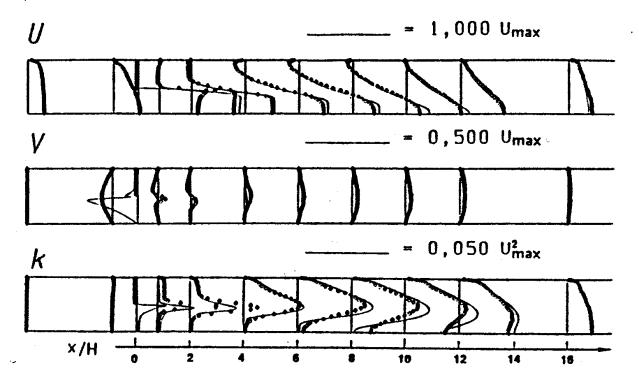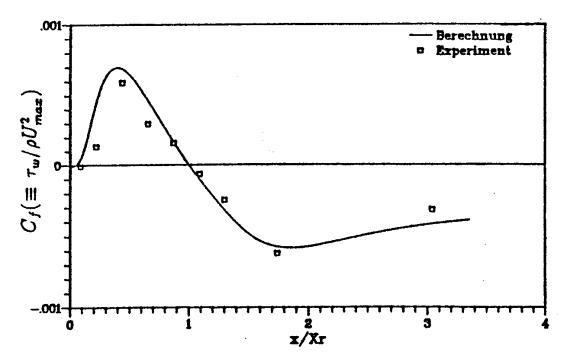# Evaluation

## Comparison of CFD calculations with Experiments

A comparison of the numerical results with the experiments was conducted for both cases A and B. The mean velocities were predicted reasonably well, however, a small over-prediction of the axial velocity in the region of the vena contracta was observed (Fig. 6). In the shear layer at x/H = 2 the turbulent kinetic energy was considerably under-predicted. Further downstream an over-prediction of the maximum k-value was found (Fig. 6). The above conclusions can be also made for case A, however, the agreement with the measurements was slightly better, especially for the turbulent kinetic energy. The calculated normalized wall friction coefficient for this case also agreed very well with the measurements (Fig. 7)Fig. 6 Comparison of computed and measured profiles of the mean velocities and the turbulent kinetic energy for case B with Re = 22,000 using 248 x 56 gridsFig. 7 Comparison of the computed and measured normalized wall friction coefficient along the pipe, starting at the orifice for case A and Re = 22,000